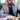# BinarySearch.io Longest Sublist with K Distinct Numbers

February 24, 2021

### Problem:

Given an integer k and a list of integers nums, return the length of the longest sublist that contains at most k distinct integers. Problem link: https://binarysearch.com/problems/Longest-Sublist-with-K-Distinct-Numbers

### Intuition:

Python Two-Pointer/Sliding-Window and Dictionary approach

Use 2 pointers `l`(left) and `r`(right) to traverse the array

### Implementation:

We use a dictionary `curr_set` to keep track of elements in the current window. When we find an element not in the current set we start popping elements from the left

### Code

``````class Solution:
def solve(self, k, nums):
if k == 0:
return 0
if not nums:
return 0
curr_set = collections.defaultdict(int)
l = 0
max_len = 0
curr_len = 0

for r in range(0, len(nums)):
while len(curr_set) >= k and nums[r] not in curr_set:
curr_set[nums[l]] -= 1
if curr_set[nums[l]] == 0:
del curr_set[nums[l]]
l += 1
curr_set[nums[r]] += 1
max_len = max(max_len, r - l + 1)
return max_len``````

O(n)

### Space complexity

O(k) where k is the size of curr_setWritten by Ganesh Iyer A software engineer building platforms for leveraging artificial intelligence in healthcare.